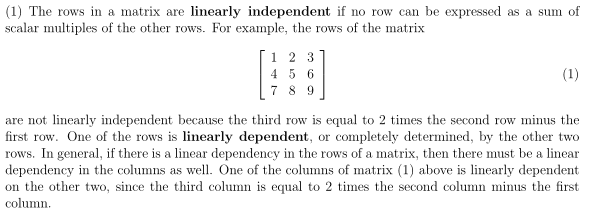# Prove of linearly dependency of rows in matrices

## Homework Statement

If the rows of A are linearly dependent, prove that the rows of AB are also linearly dependent.

## The Attempt at a Solution

$$A = \begin{pmatrix}a&-a\\b&-b\end{pmatrix}$$ the rows are linearly dependent because a - a = 0 and b - b = 0.

$$B = \begin{pmatrix}c_1&c_2\\c_3&c_4\end{pmatrix}$$

Then$$AB = \begin{pmatrix}a(c_1-c_3)&a(c_2-c_4)\\b(c_1-c_3)&b(c_2-c_4)\end{pmatrix} \; where\; c_1 \neq c_3\; and\; c_2 \neq c_4$$

But then wouldn't these rows now be linearly independent? Unless $$c_1 - c_3 = -(c_2 - c_4)$$

Thanks for any help.

can you show dependence (or the opposite?) by saying that the det(AB) ≠ 0 ?

i don't have my materials in front of me to check to see which is true. . .

can you show dependence (or the opposite?) by saying that the det(AB) ≠ 0 ?

i don't have my materials in front of me to check to see which is true. . .

Yeah I thought about going that route but it's asking for the rows not the matrix being linearly dependent. At least that's what I hope I'm understanding of this question.so i guess show that the rows are multiples of each other. . .

That paragraph actually does help me a lot because the determinant can be used to prove columns of the matrix are linearly dependent and then that paragraph states if the rows are dependent then so are the columns. I would assume that the inverse is also true to help my case. I calculated the determinant and it was 0.

That paragraph actually does help me a lot because the determinant can be used to prove columns of the matrix are linearly dependent and then that paragraph states if the rows are dependent then so are the columns. I would assume that the inverse is also true to help my case. I calculated the determinant and it was 0.

Yes, you can use the determinant for this. A matrix has nonzero determinant if and only if the set of row vectors and the set of column vectors form linearly independent sets. Assuming you have proved (or it has been stated) in class
$\det{A}\det{B}=\det{AB}$, I would recommend you take this route.

Ray Vickson
Homework Helper
Dearly Missed
Yes, you can use the determinant for this. A matrix has nonzero determinant if and only if the set of row vectors and the set of column vectors form linearly independent sets. Assuming you have proved (or it has been stated) in class
$\det{A}\det{B}=\det{AB}$, I would recommend you take this route.

In general, A, B and AB may be non-square and so not have determinants. Nevertheless the stated result is still true. In fact, it is pretty easy, but I'll leave you to have the fun of doing it.

RGV

Mark44
Mentor

## Homework Statement

If the rows of A are linearly dependent, prove that the rows of AB are also linearly dependent.

## The Attempt at a Solution

$$A = \begin{pmatrix}a&-a\\b&-b\end{pmatrix}$$ the rows are linearly dependent because a - a = 0 and b - b = 0.
???
Are you given that A and B are 2x2 matrices? If so, for the rows of a 2x2 matrix to be dependent, they must be multiples of each other. In the matrix you show, the first row is a multiple of <1, -1>. The second row is also a multiple of <1, -1>.
$$B = \begin{pmatrix}c_1&c_2\\c_3&c_4\end{pmatrix}$$

Then$$AB = \begin{pmatrix}a(c_1-c_3)&a(c_2-c_4)\\b(c_1-c_3)&b(c_2-c_4)\end{pmatrix} \; where\; c_1 \neq c_3\; and\; c_2 \neq c_4$$

But then wouldn't these rows now be linearly independent? Unless $$c_1 - c_3 = -(c_2 - c_4)$$

Thanks for any help.